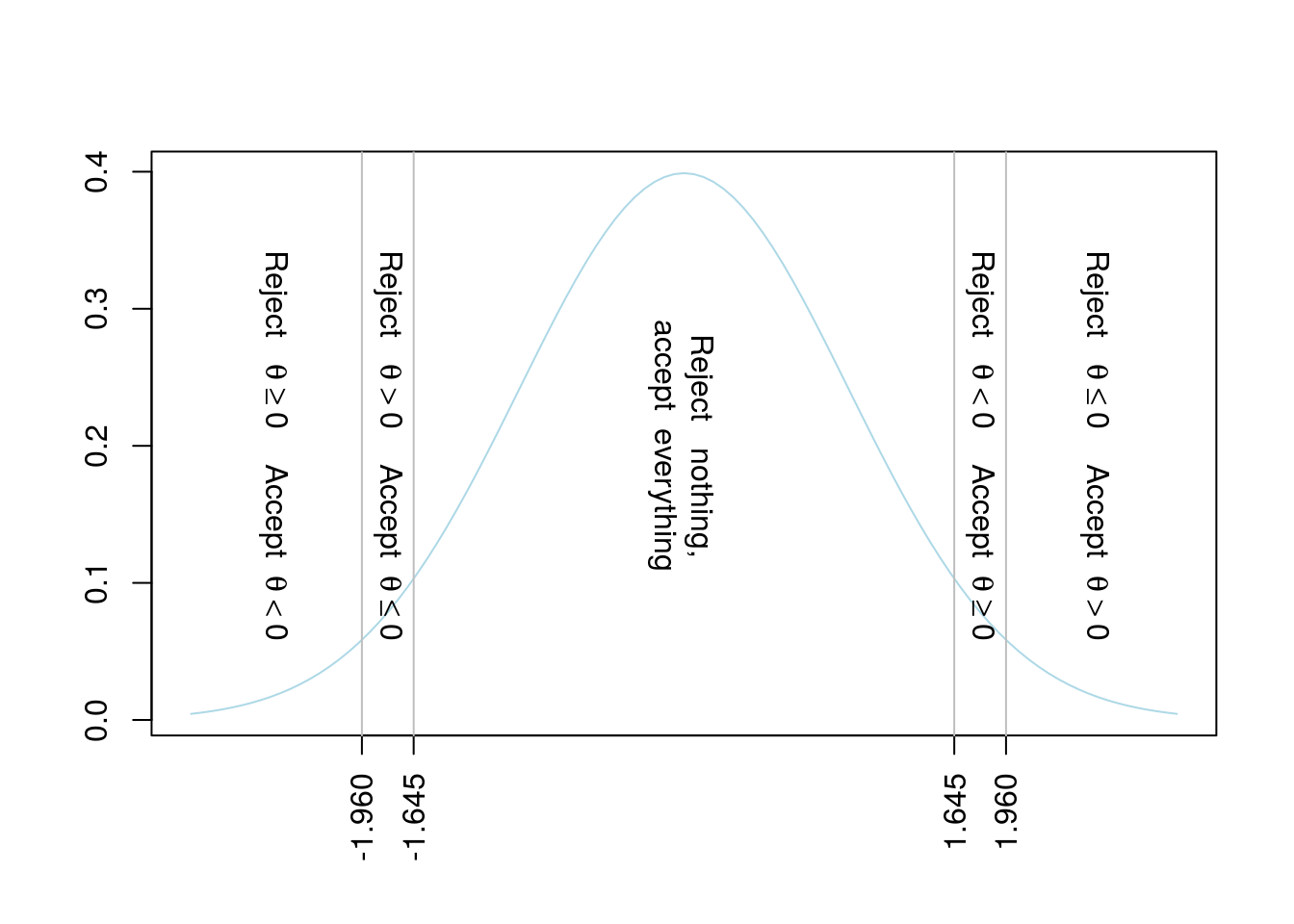# Aaron McDaid

Statistics and C++, and other fun projects

aaron.mcdaid@gmail.com

@aaronmcdaid

by Aaron, 30th October 2017

(code for this document)

This is a short post summarising some recent work (arXiv link) I did with Zoltan Kutalik and Valentin Rousson

# The null hypothesis

Given a sample of, say, $$n=100$$ observations from a Normal distribution with variance 100, we wish to make inferences about the mean, $$\theta$$,

$x_i \sim \mathcal{N}(\theta,100)$

For simplicity, assume that we really just want to know which is these three are true:

1. $$\theta < 0$$
2. $$\theta = 0$$
3. $$\theta > 0$$

We compute the sample mean from our 100 observations,

$\bar{x} = \frac1n \sum_{i=1}^n x_i$

If the sample mean is large, e.g. $$\bar{x} > 10$$, then it is virtually certain that $$\theta > 0$$ and we can safely reject the other two hypotheses. This is because, if $$\theta{\le}0$$, the probability of seeing $$\bar{x} > 10$$ is very small, less than $$7 \times 10^{-24}$$.

variance.of.one.observation = 100
n=100
variance.of.sample.mean = variance.of.one.observation / n
prob.of.sample.mean.at.least.10 = pnorm(10, mean=0, sd=sqrt(variance.of.sample.mean), lower.tail=F)

PP(n, variance.of.sample.mean, prob.of.sample.mean.at.least.10)
##                              n  =  100
##        variance.of.sample.mean  =  1
## prob.of.sample.mean.at.least.10  =  7.61985302416053e-24

Similarly, if $$\bar{x} < -10$$, then it is virtually certain that $$\theta > 0$$ and we can safely reject the other two hypotheses.

# Error rates

If our sample mean is close to zero however, what to we do? We need to set thresholds, where we accept our reject the hypotheses depending on the value of the sample mean. It is common to decide these thresholds by first fixing an error rate, typically $$\alpha = 0.05$$, and then calculating the thresholds such that the probability of incorrectly rejecting a hypothesis is no more than 5%.

We propose dividing the possible sample means into five bins. As far as we are aware, this particular procedure has not been proposed before. We accept or reject according to this scheme, depending on where $$\bar{x}$$ is relative to the four ‘special’ values, $$-1.96, -1.64, 1.64, 1.96$$. Look up your sample mean on the x-axis of the plot below, and then look up the reject/accept rule written in that window. There are five windows in this plot, hence the “five-decision rule”.

outer.threshold = qnorm(0.025, mean=0, sd=sqrt(variance.of.sample.mean), lower.tail=F)
inner.threshold = qnorm(0.05 , mean=0, sd=sqrt(variance.of.sample.mean), lower.tail=F)
PP(outer.threshold, inner.threshold)
##                outer.threshold  =  1.95996398454005
##                inner.threshold  =  1.64485362695147To prove that the error rate is at most 5%, we consider each of the three possible “truths” in turn:

Possibility 1/3: If $$\theta<0$$, then only the last two bins, when $$\bar{x}>1.64$$, constitute an error. For any $$\theta<0$$, the probability of observing $$\bar{x}>1.64$$ is at most 5%. Of course, for $$\theta$$ which is truly much smaller than zero, the probability of error will be much smaller than 5%.

Possibility 2/3: If $$\theta=0$$, then only the outer two bins, when $$|\bar{x}|>1.96$$, constitute an error. And the probability, assuming $$\theta=0$$, of observing $$|\bar{x}|>1.96$$ is 5%.

Possibility 3/3: If $$\theta>0$$, then only the first two bins, when $$\bar{x}<-1.64$$, constitute an error. For any $$\theta>0$$, the probability of observing $$\bar{x}<-1.64$$ is at most 5%. Of course, for $$\theta$$ which is truly much greater than zero, the probability of error will be much smaller than 5%.

# Improved power

We propose this method because it is more powerful than existing methods. A simple two-sided test will simply consider two possibilities, either $$\theta=0$$ or $$\theta \not= 0$$, rejecting the former if $$|\bar{x}|>1.96$$. A slightly more powerful procedure than this two-sided test is to infer the direction of the test statistic also. When $$|\bar{x}|>1.96$$ and we have rejected $$\theta \not= 0$$, we are entitled to look at the sign of $$\bar{x}$$ also to reject $$\theta \le 0$$ or $$\theta \ge 0$$.

Our procedure is more powerful than these approaches, as we also make rejections when $$1.65<|\bar{x}|<1.96$$. We are able to make these rejections without requiring any extra assumptions - in advance - about the direction.

Remember however, that these ‘extra’ rejections are relatively weak rejections. Depending on the application, rejecting $$\theta<0$$ and accepting that the null hypothesis might not be very helpful. But it might be useful when testing the side effects of a drug. We are satisfied in showing the effects are not negative - it is not always necessary to show a positive effect of the drug on every aspect of the patients life.

We do not claim this replaces the conventional one-sided test in all cases. That test is very useful when we have a clear idea, in advance, of which direction is more “interesting” to reject.

# Impossible null

It’s beyond the scope of this short post, but there are contexts where the null hypothesis is believed to be impossible before any experiment is performed. Some would argue that every drug has a non-zero effect, even though it might be very close to zero. If you believe this, in the context of your experiment, then our test simplifies to be equivalent to the three-decision testing procedure of Jones and Tukey (2000).

# Extending this

The above can be extended in obvious ways to other distributions and other error rates, as long as you can calculate the relevant threholds at your desired error rates.

by Aaron, 29th October 2017